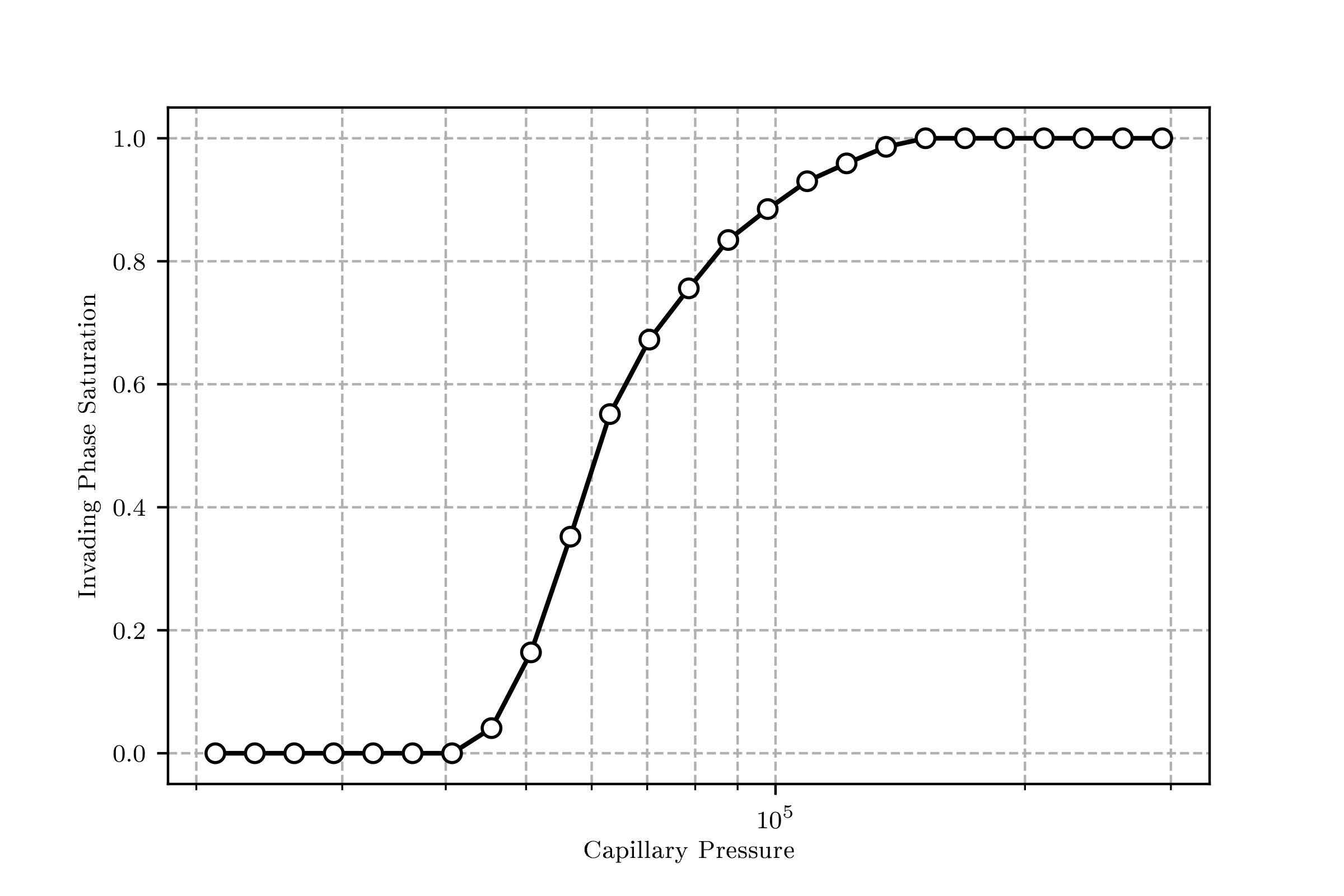# Basic Usage¶

It is relatively easy to get going with a quick simulation in OpenPNM. In fact the following code block produces a mercury intrusion simulation in just a few lines.

## Problem setup¶

```import openpnm as op

# Define geometrical parameters
Lc = 1e-4
Nx, Ny, Nz = (10, 10, 10)

# Generate network, geometry, phase, and physics
pn = op.network.Cubic(shape=[Nx, Ny, Nz], spacing=Lc)
geo = op.geometry.SpheresAndCylinders(network=pn, pores=pn.Ps, throats=pn.Ts)
Hg = op.phases.Mercury(network=pn)
phys = op.physics.Standard(network=pn, phase=Hg, geometry=geo)

# Create algorithm and run simulation
mip = op.algorithms.Porosimetry(network=pn, phase=Hg)
mip.set_inlets(pores=pn.pores(['left', 'right', 'top', 'bottom']))
mip.run()
```

## Basic visualization¶

The results can be visualized with `mip.plot_intrusion_curve()` giving something like this:## Calculating permeability¶

As another example, the permeability coefficient can be found as follows:

```# Generate phase and physics
water = op.phases.Water(network=pn)
phys = op.physics.Standard(network=pn, phase=water, geometry=geo)

# Create algorithm, set boundary conditions and run simulation
sf = op.algorithms.StokesFlow(network=pn, phase=water)
Pin, Pout = (200_000, 101_325)
sf.set_value_BC(pores=pn.pores('left'), values=Pin)
sf.set_value_BC(pores=pn.pores('right'), values=Pout)
sf.run()
```

The total flow rate into the domain through the boundary pores can be found using `sf.rate(pores=pn.pores('left'))`. The permeability coefficient can be found by inserting known values into Darcy’s law as follows:

```Q = sf.rate(pores=pn.pores('left'))
A = Ny*Nz*Lc**2
L = Nx*Lc
mu = water['pore.viscosity'].mean()
K = Q*mu*L/(A*(Pin-Pout))
```

It’s also worth explaining how to adjust the pore size distribution of the network, so that the capillary curve and permeability coefficient can be changed to match known values. The `geo` object controls the geometric properties, and it possess models to calculate values on demand. Let’s change the pore size distribution to a Weibull distribution, but first let’s store the existing values in a dummy variable so we can compare later.

```import op.models.geometry as gmods

geo['pore.old_diameter'] = geo.pop('pore.diameter')
Now you can run `geo.show_hist(['pore.old_diameter', 'pore.diameter'])` to get a quick glance at the histograms of the two distributions.